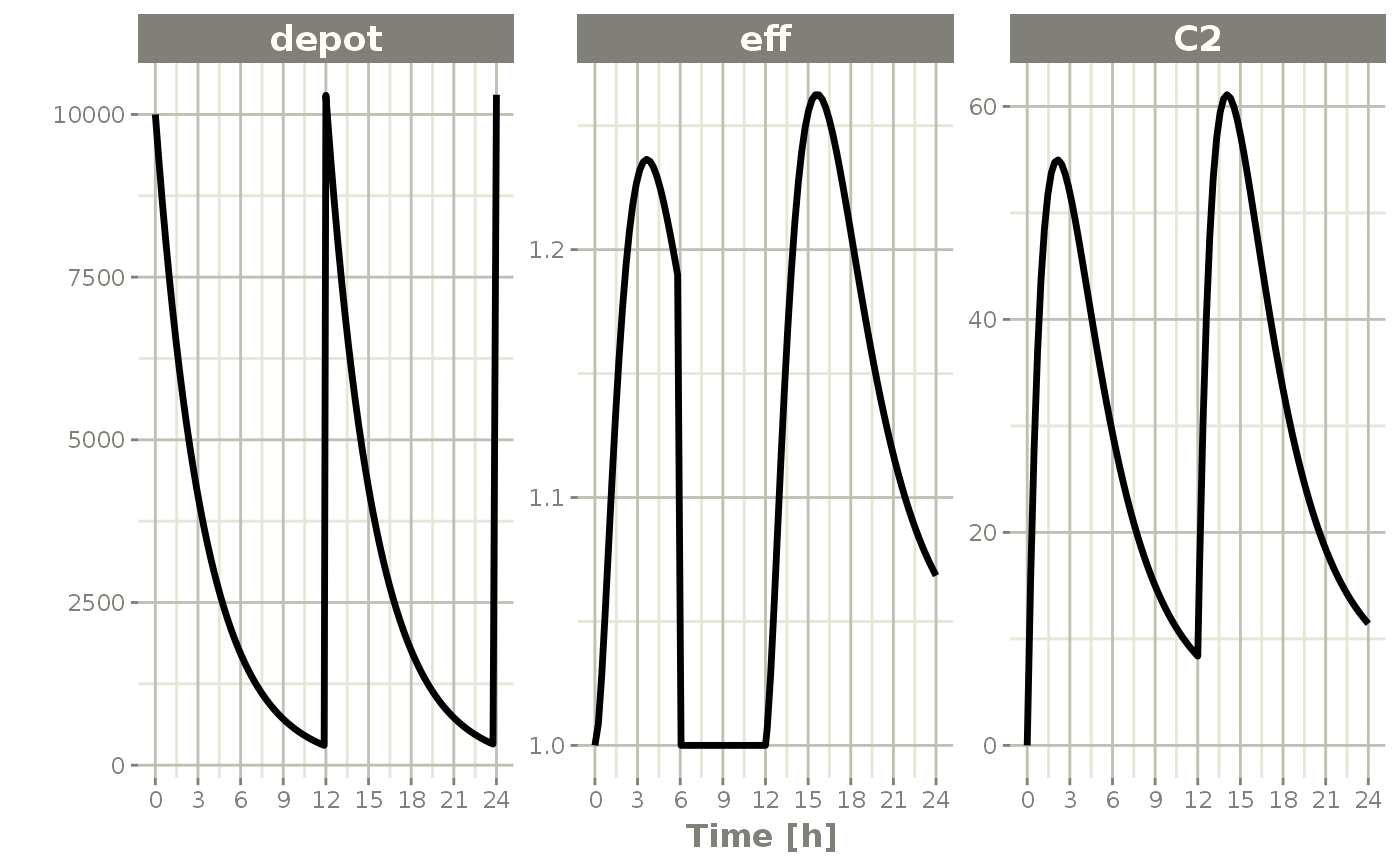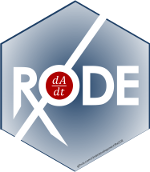RxODE event tables

In general, RxODE event tables follow NONMEM dataset convention with the exceptions:

• The compartment data item (cmt) can be a string/factor with compartment names
• You may turn off a compartment with a negative compartment number or “-cmt” where cmt is the compartment name.
• The compartment data item (cmt) can still be a number, the number of the compartment is defined by the appearance of the compartment name in the model. This can be tedious to count, so you can specify compartment numbers easier by using the cmt(cmtName) at the beginning of the model.
• An additional column, dur can specify the duration of infusions;
• Bioavailability changes will change the rate of infusion since dur/amt are fixed in the input data.
• Similarly, when specifying rate/amt for an infusion, the bioavailability will change the infusion duration since rate/amt are fixed in the input data.
• Some infrequent NONMEM columns are not supported: pcmt, call.
• evid=5 or replace event; This replaces the value of a compartment with the value specified in the amt column. This is equivalent to deSolve=replace.
• evid=6 or multiply event; This multiplies the value in the compartment with the value specified by the amt column. This is equivalent to deSolve=multiply.

Here are the legal entries to a data table:

Data Item Meaning Notes
id Individual identifier Can be a integer, factor, character, or numeric
time Individual time Numeric for each time.
amt dose amount Positive for doses zero/NA for observations
rate infusion rate When specified the infusion duration will be dur=amt/rate
rate = -1, rate modeled; rate = -2, duration modeled
dur infusion duration When specified the infusion rate will be rate = amt/dur
evid event ID 0=Observation; 1=Dose; 2=Other; 3=Reset; 4=Reset+Dose; 5=Replace; 6=Multiply
cmt Compartment Represents compartment #/name for dose/observation
ii Inter-dose Interval Time between doses.
addl # of additional doses Number of doses like the current dose.

Other notes:

• The evid can be the classic RxODE (described here) or the NONMEM-style evid described above.
• NONMEM’s DV is not required; RxODE is a ODE solving framework.
• NONMEM’s MDV is not required, since it is captured in EVID.
• Instead of NONMEM-compatible data, it can accept deSolve compatible data-frames.

When returning the RxODE solved data-set there are a few additional event ids (EVID) that you may see depending on the solving options:

• EVID = -1 is when a modeled rate ends (corresponds to rate = -1)
• EVID = -2 is when a modeled duration ends (corresponds to rate=-2)
• EVID = -10 when a rate specified zero-order infusion ends (corresponds to rate > 0)
• EVID = -20 when a duration specified zero-order infusion ends (corresponds to dur > 0)
• EVID = 101, 102, 103,... These correspond to the 1, 2, 3, … modeled time (mtime).

These can only be accessed when solving with the option combination addDosing=TRUE and subsetNonmem=FALSE. If you want to see the classic EVID equivalents you can use addDosing=NA.

To illustrate the event types we will use the model from the original RxODE tutorial.

#>   no cache: create with rxCreateCache()
## Model from RxODE tutorial
m1 <-RxODE({
KA=2.94E-01;
CL=1.86E+01;
V2=4.02E+01;
Q=1.05E+01;
V3=2.97E+02;
Kin=1;
Kout=1;
EC50=200;
## Added modeled bioavaiblity, duration and rate
fdepot = 1;
durDepot = 8;
rateDepot = 1250;
C2 = centr/V2;
C3 = peri/V3;
d/dt(depot) =-KA*depot;
f(depot) = fdepot
dur(depot) = durDepot
rate(depot) = rateDepot
d/dt(centr) = KA*depot - CL*C2 - Q*C2 + Q*C3;
d/dt(peri)  =                    Q*C2 - Q*C3;
d/dt(eff)  = Kin - Kout*(1-C2/(EC50+C2))*eff;
eff(0) = 1
});

A bolus dose is the default type of dose in RxODE and only requires the amt/dose. Note that this uses the convenience function et() described in the RxODE event tables

ev <- et(timeUnits="hr") %>%
et(amt=10000, ii=12,until=24) %>%
et(seq(0, 24, length.out=100))

ev
#> -------------------------- EventTable with 101 records -------------------------
#>
#>    1 dosing records (see x$get.dosing(); add with add.dosing or et) #> 100 observation times (see x$get.sampling(); add with add.sampling or et)
#>    multiple doses in addl columns, expand with x$expand(); or etExpand(x) #> -- First part of x: ------------------------------------------------------------ #> # A tibble: 101 x 5 #> time amt ii addl evid #> [h] <dbl> [h] <int> <evid> #> 1 0 NA NA NA 0:Observation #> 2 0 10000 12 2 1:Dose (Add) #> 3 0.242 NA NA NA 0:Observation #> 4 0.485 NA NA NA 0:Observation #> 5 0.727 NA NA NA 0:Observation #> 6 0.970 NA NA NA 0:Observation #> 7 1.21 NA NA NA 0:Observation #> 8 1.45 NA NA NA 0:Observation #> 9 1.70 NA NA NA 0:Observation #> 10 1.94 NA NA NA 0:Observation #> # ... with 91 more rows rxSolve(m1, ev) %>% plot(C2) + xlab("Time")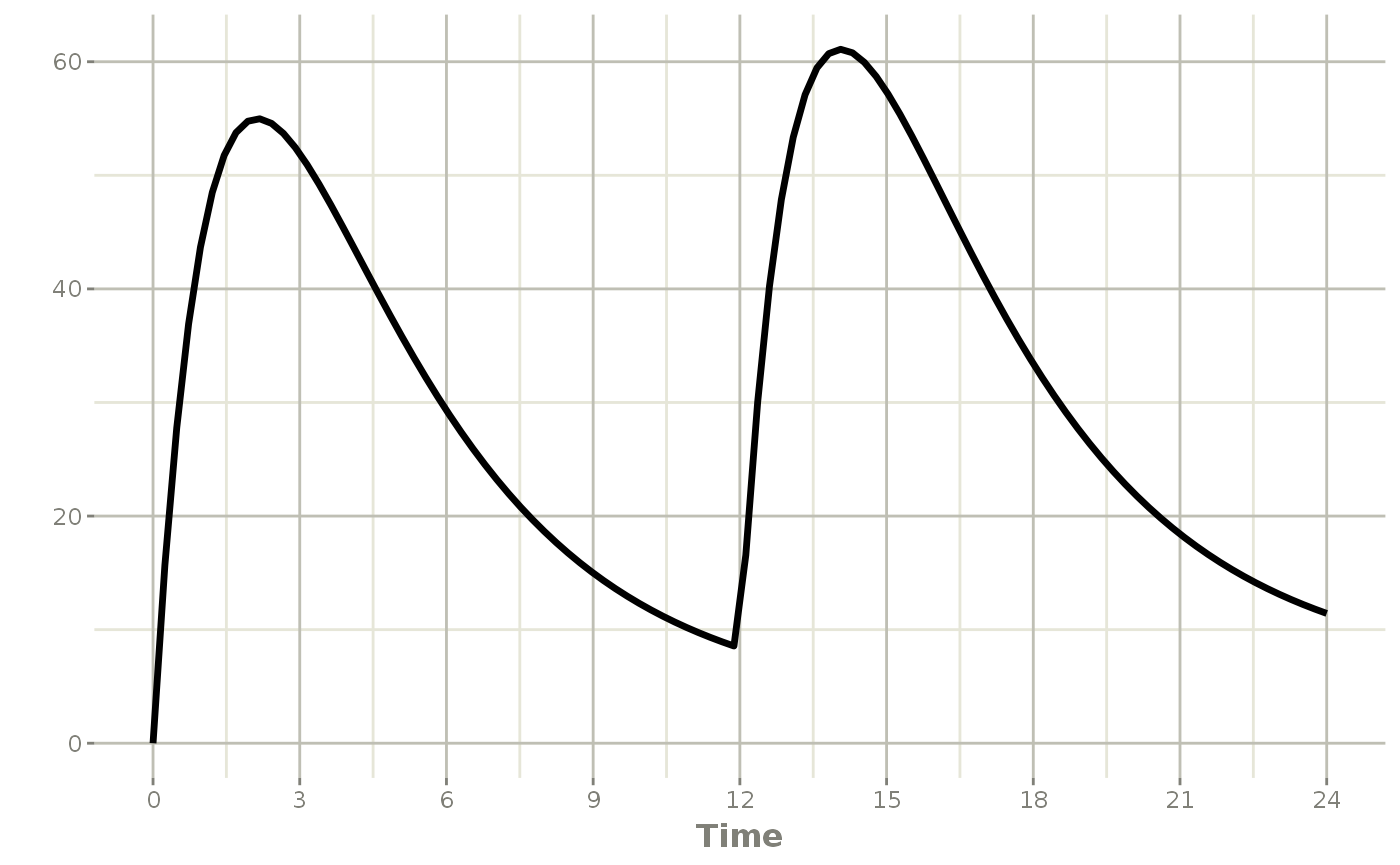Infusion Doses There are a few different type of infusions that RxODE supports: • Constant Rate Infusion (rate) • Constant Duration Infusion (dur) • Estimated Rate of Infusion • Estimated Duration of Infusion Constant Infusion (in terms of duration and rate) The next type of event is an infusion; There are two ways to specify an infusion; The first is the dur keyword. An example of this is: ev <- et(timeUnits="hr") %>% et(amt=10000, ii=12,until=24, dur=8) %>% et(seq(0, 24, length.out=100)) ev #> -------------------------- EventTable with 101 records ------------------------- #> #> 1 dosing records (see x$get.dosing(); add with add.dosing or et)
#>    100 observation times (see x$get.sampling(); add with add.sampling or et) #> multiple doses in addl columns, expand with x$expand(); or etExpand(x)
#> -- First part of x: ------------------------------------------------------------
#> # A tibble: 101 x 6
#>     time   amt    ii  addl evid            dur
#>      [h] <dbl>   [h] <int> <evid>          [h]
#>  1 0        NA    NA    NA 0:Observation    NA
#>  2 0     10000    12     2 1:Dose (Add)      8
#>  3 0.242    NA    NA    NA 0:Observation    NA
#>  4 0.485    NA    NA    NA 0:Observation    NA
#>  5 0.727    NA    NA    NA 0:Observation    NA
#>  6 0.970    NA    NA    NA 0:Observation    NA
#>  7 1.21     NA    NA    NA 0:Observation    NA
#>  8 1.45     NA    NA    NA 0:Observation    NA
#>  9 1.70     NA    NA    NA 0:Observation    NA
#> 10 1.94     NA    NA    NA 0:Observation    NA
#> # ... with 91 more rows
rxSolve(m1, ev) %>% plot(depot, C2) +
xlab("Time")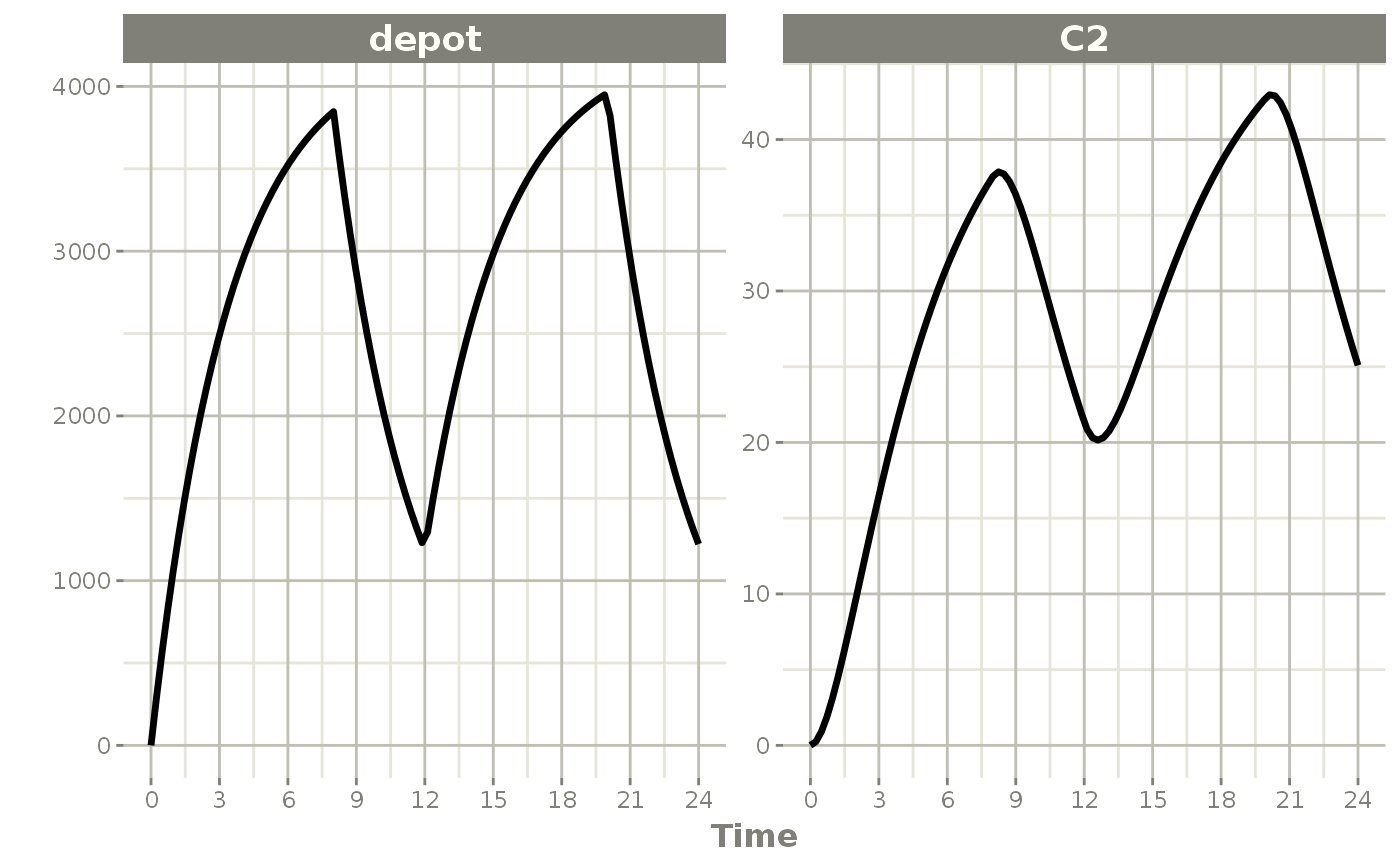It can be also specified by the rate component:

ev <- et(timeUnits="hr") %>%
et(amt=10000, ii=12,until=24, rate=10000/8) %>%
et(seq(0, 24, length.out=100))

ev
#> -------------------------- EventTable with 101 records -------------------------
#>
#>    1 dosing records (see x$get.dosing(); add with add.dosing or et) #> 100 observation times (see x$get.sampling(); add with add.sampling or et)
#>    multiple doses in addl columns, expand with x$expand(); or etExpand(x) #> -- First part of x: ------------------------------------------------------------ #> # A tibble: 101 x 6 #> time amt rate ii addl evid #> [h] <dbl> <rate/dur> [h] <int> <evid> #> 1 0 NA NA NA NA 0:Observation #> 2 0 10000 1250 12 2 1:Dose (Add) #> 3 0.242 NA NA NA NA 0:Observation #> 4 0.485 NA NA NA NA 0:Observation #> 5 0.727 NA NA NA NA 0:Observation #> 6 0.970 NA NA NA NA 0:Observation #> 7 1.21 NA NA NA NA 0:Observation #> 8 1.45 NA NA NA NA 0:Observation #> 9 1.70 NA NA NA NA 0:Observation #> 10 1.94 NA NA NA NA 0:Observation #> # ... with 91 more rows rxSolve(m1, ev) %>% plot(depot, C2) + xlab("Time")These are the same with the exception of how bioavailability changes the infusion. In the case of modeling rate, a bioavailability decrease, decreases the infusion duration, as in NONMEM. For example: rxSolve(m1, ev, c(fdepot=0.25)) %>% plot(depot, C2) + xlab("Time")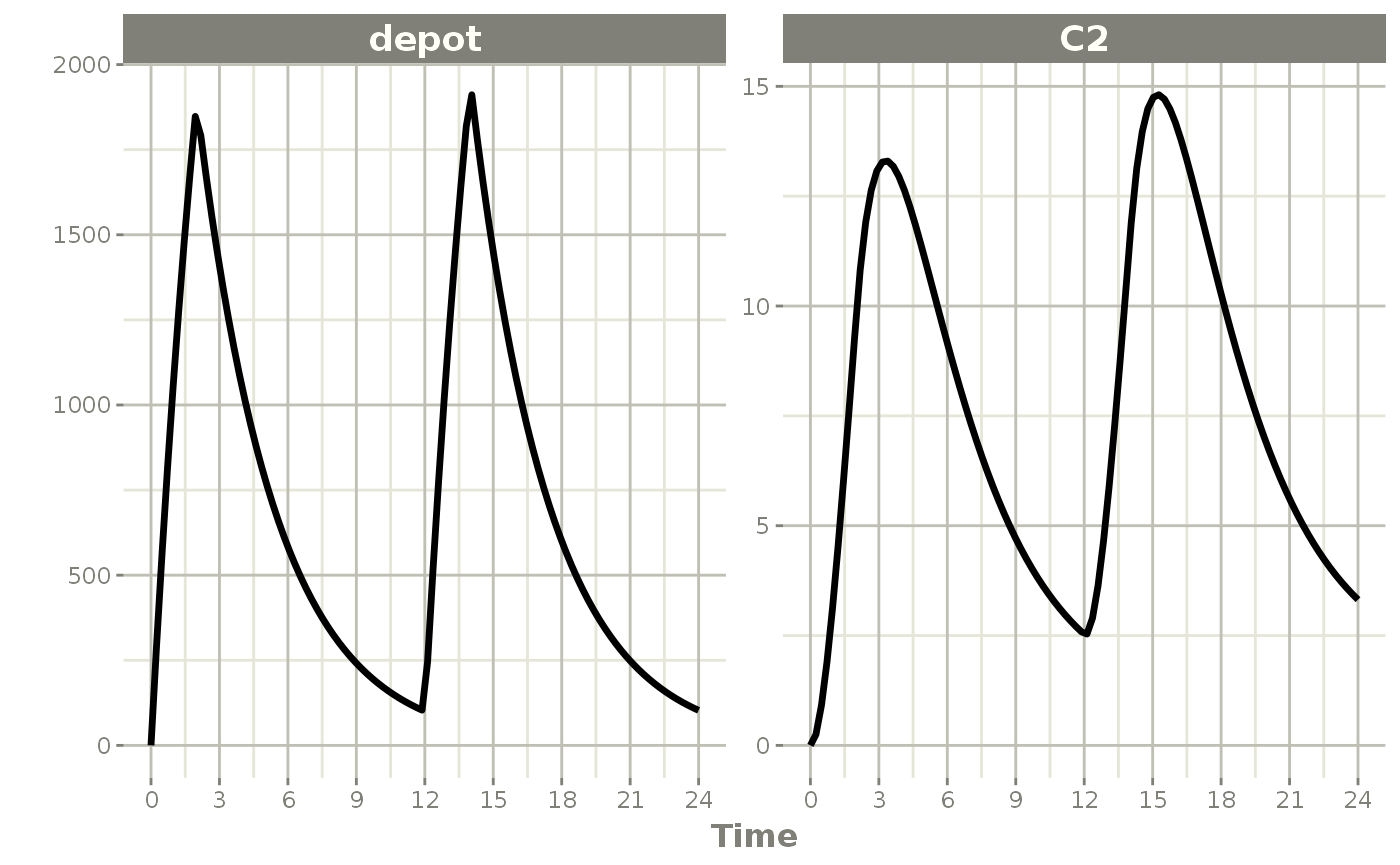Similarly increasing the bioavailability increases the infusion duration. rxSolve(m1, ev, c(fdepot=1.25)) %>% plot(depot, C2) + xlab("Time")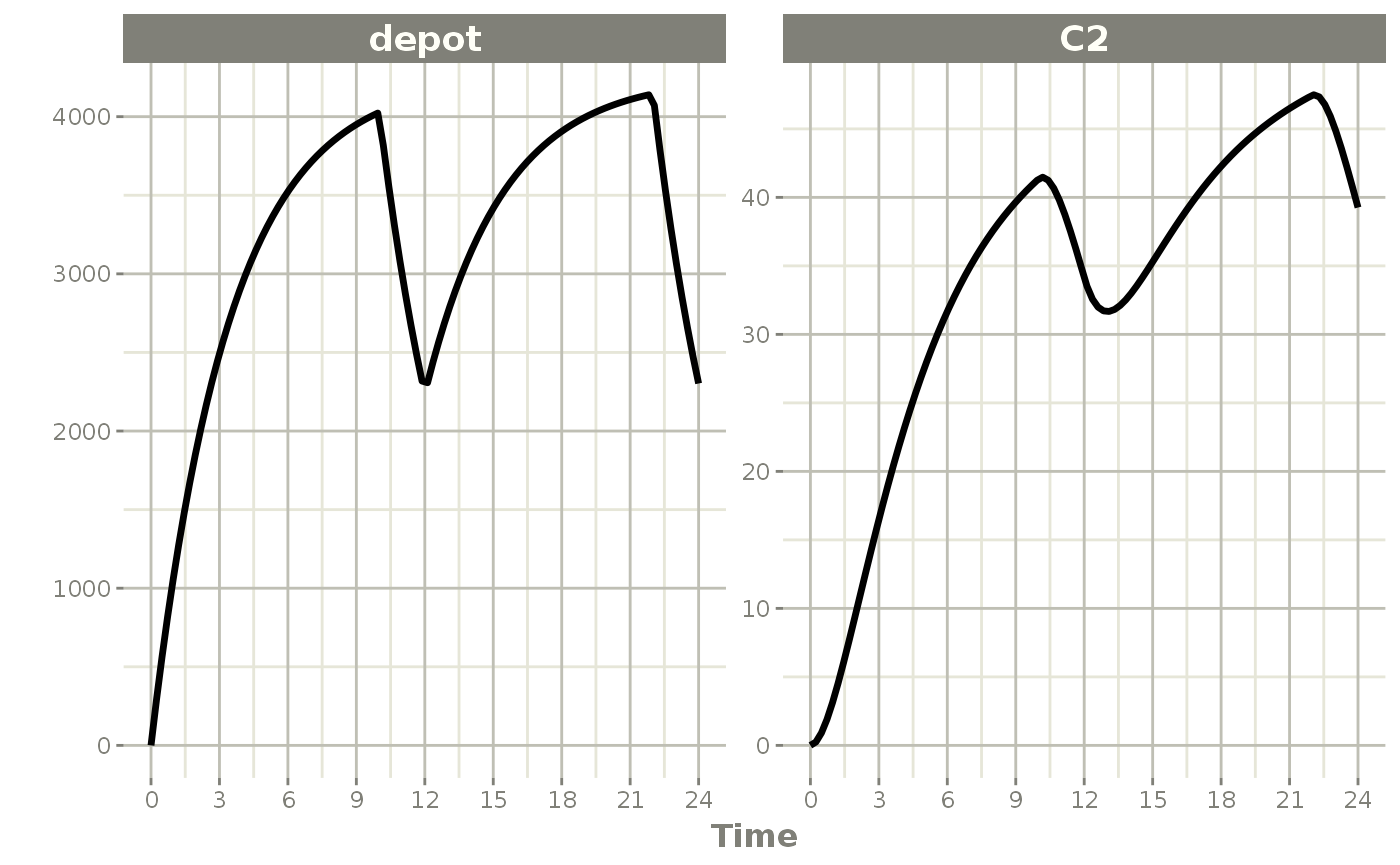The rationale for this behavior is that the rate and amt are specified by the event table, so the only thing that can change with a bioavailability increase is the duration of the infusion. If you specify the amt and dur components in the event table, bioavailability changes affect the rate of infusion. ev <- et(timeUnits="hr") %>% et(amt=10000, ii=12,until=24, dur=8) %>% et(seq(0, 24, length.out=100)) You can see the side-by-side comparison of bioavailability changes affecting rate instead of duration with these records in the following plots: library(ggplot2) library(patchwork) p1 <- rxSolve(m1, ev, c(fdepot=1.25)) %>% plot(depot) + xlab("Time") + ylim(0,5000) p2 <- rxSolve(m1, ev, c(fdepot=0.25)) %>% plot(depot) + xlab("Time")+ ylim(0,5000) ## Use patchwork syntax to combine plots p1 * p2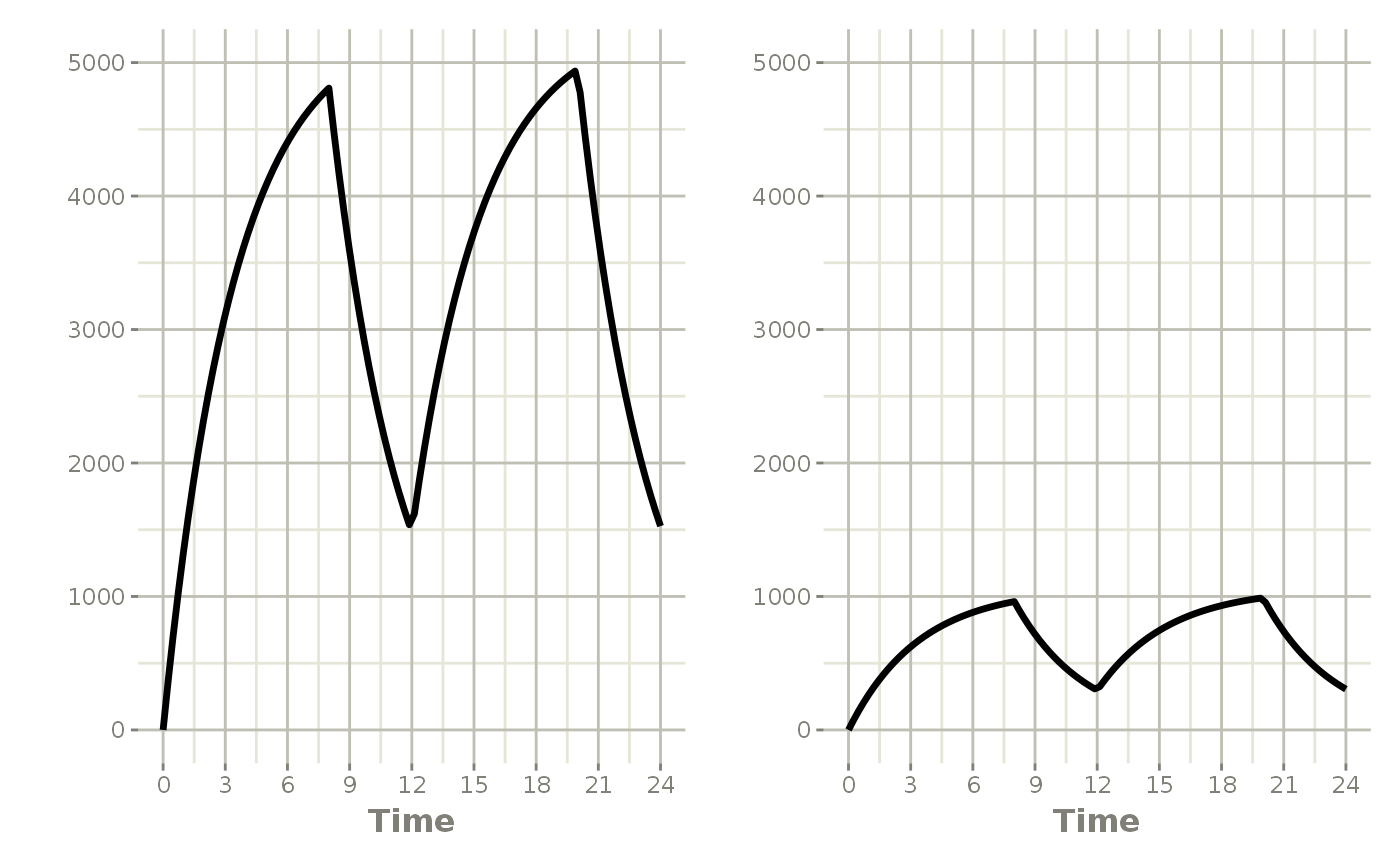Modeled Rate and Duration of Infusion You can model the duration, which is equivalent to NONMEM’s rate=-2. As a mnemonic you can use the dur=model instead of rate=-2 ev <- et(timeUnits="hr") %>% et(amt=10000, ii=12,until=24, dur=model) %>% et(seq(0, 24, length.out=100)) ev #> -------------------------- EventTable with 101 records ------------------------- #> #> 1 dosing records (see x$get.dosing(); add with add.dosing or et)
#>    100 observation times (see x$get.sampling(); add with add.sampling or et) #> multiple doses in addl columns, expand with x$expand(); or etExpand(x)
#> -- First part of x: ------------------------------------------------------------
#> # A tibble: 101 x 6
#>     time   amt rate          ii  addl evid
#>      [h] <dbl> <rate/dur>   [h] <int> <evid>
#>  1 0        NA NA            NA    NA 0:Observation
#>  2 0     10000 -2:dur        12     2 1:Dose (Add)
#>  3 0.242    NA NA            NA    NA 0:Observation
#>  4 0.485    NA NA            NA    NA 0:Observation
#>  5 0.727    NA NA            NA    NA 0:Observation
#>  6 0.970    NA NA            NA    NA 0:Observation
#>  7 1.21     NA NA            NA    NA 0:Observation
#>  8 1.45     NA NA            NA    NA 0:Observation
#>  9 1.70     NA NA            NA    NA 0:Observation
#> 10 1.94     NA NA            NA    NA 0:Observation
#> # ... with 91 more rows
rxSolve(m1, ev, c(durDepot=7)) %>% plot(depot, C2) +
xlab("Time")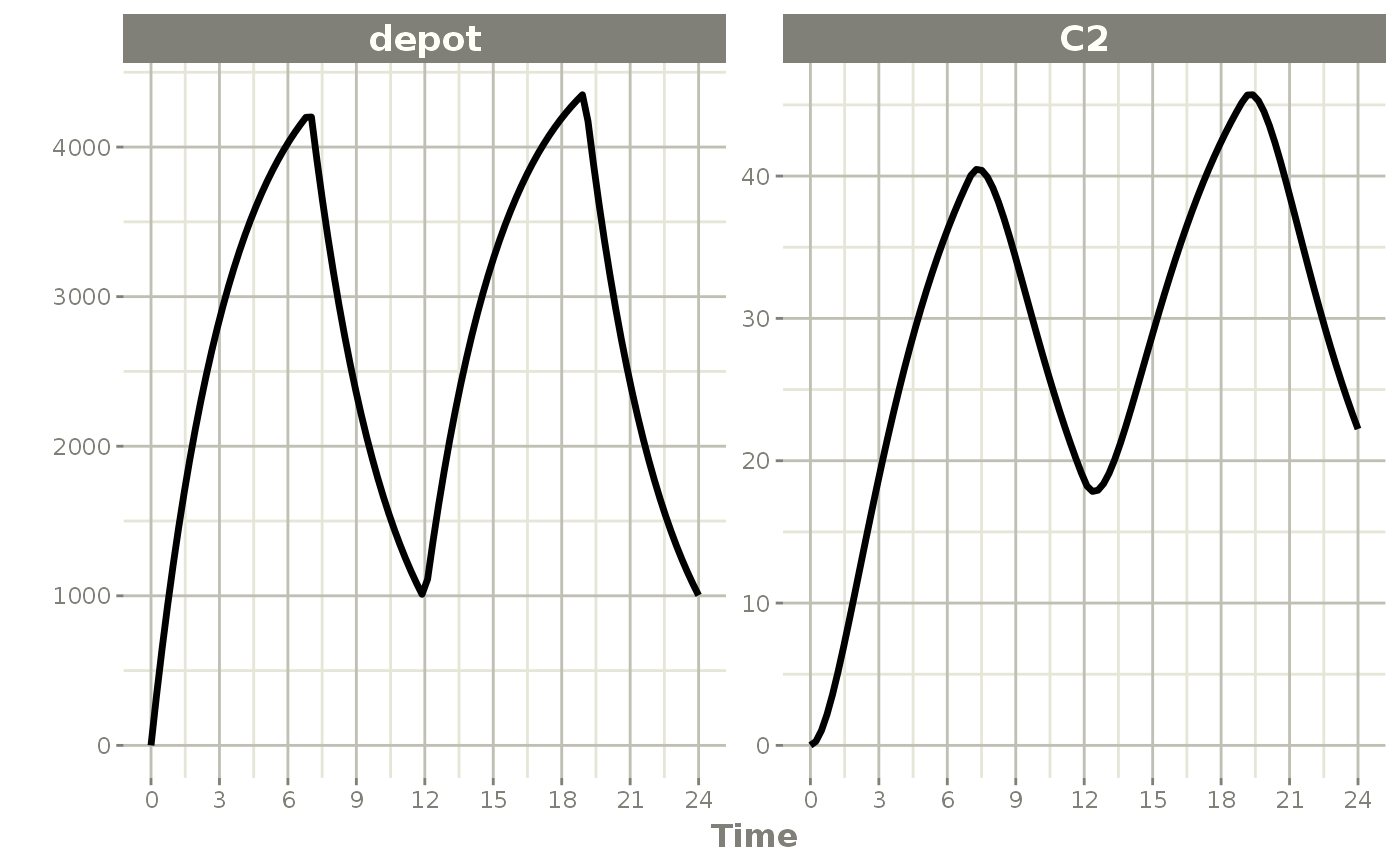Similarly, you may also model rate. This is equivalent to NONMEM’s rate=-1 and is how RxODE’s event table specifies the data item as well. You can also use rate=model as a mnemonic:

ev <- et(timeUnits="hr") %>%
et(amt=10000, ii=12,until=24, rate=model) %>%
et(seq(0, 24, length.out=100))

ev
#> -------------------------- EventTable with 101 records -------------------------
#>
#>    1 dosing records (see x$get.dosing(); add with add.dosing or et) #> 100 observation times (see x$get.sampling(); add with add.sampling or et)
#>    multiple doses in addl columns, expand with x$expand(); or etExpand(x) #> -- First part of x: ------------------------------------------------------------ #> # A tibble: 101 x 6 #> time amt rate ii addl evid #> [h] <dbl> <rate/dur> [h] <int> <evid> #> 1 0 NA NA NA NA 0:Observation #> 2 0 10000 -1:rate 12 2 1:Dose (Add) #> 3 0.242 NA NA NA NA 0:Observation #> 4 0.485 NA NA NA NA 0:Observation #> 5 0.727 NA NA NA NA 0:Observation #> 6 0.970 NA NA NA NA 0:Observation #> 7 1.21 NA NA NA NA 0:Observation #> 8 1.45 NA NA NA NA 0:Observation #> 9 1.70 NA NA NA NA 0:Observation #> 10 1.94 NA NA NA NA 0:Observation #> # ... with 91 more rows rxSolve(m1, ev, c(rateDepot=10000/3)) %>% plot(depot, C2) + xlab("Time")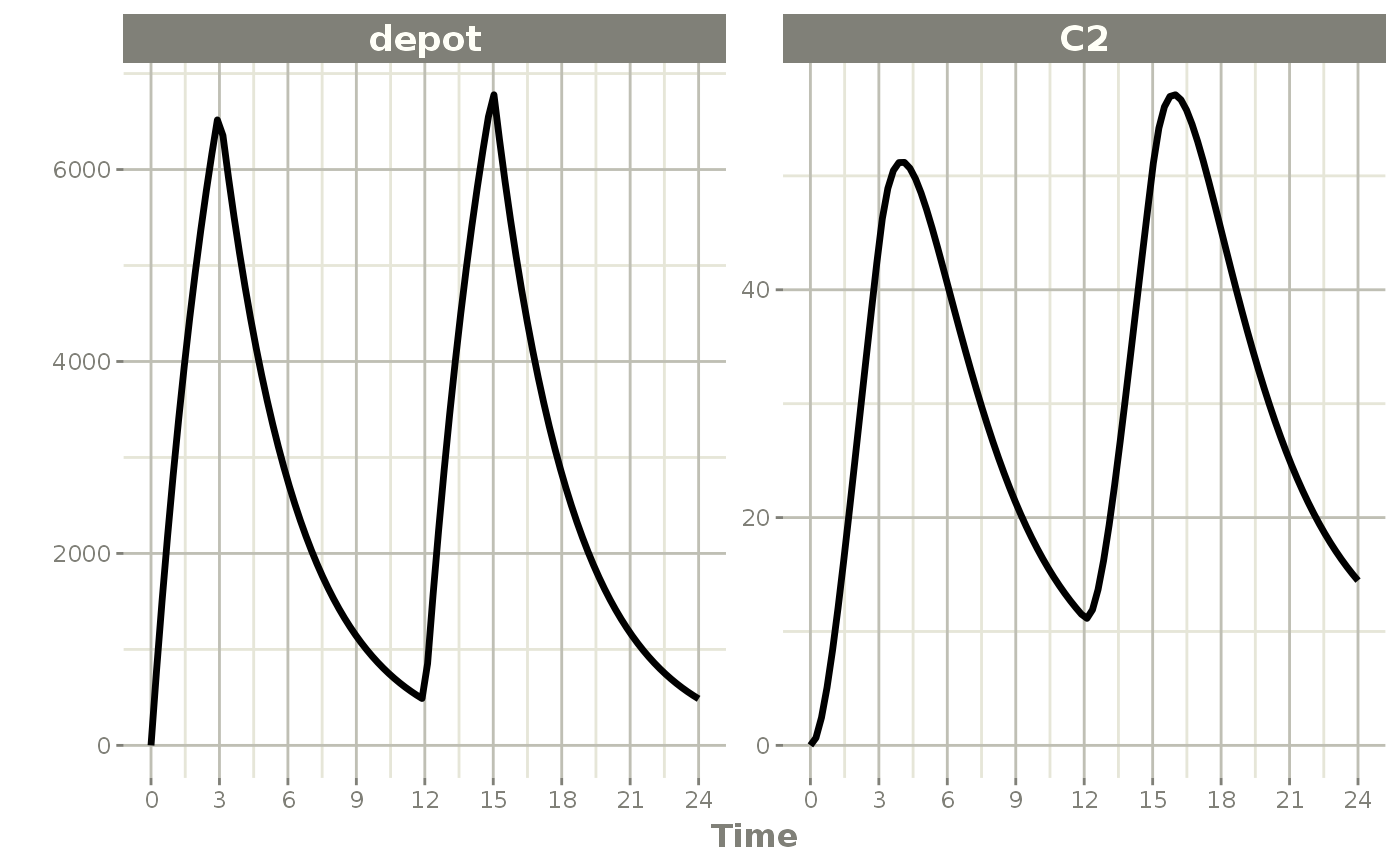Steady State These doses are solved until a steady state is reached with a constant inter-dose interval. ev <- et(timeUnits="hr") %>% et(amt=10000, ii=12, ss=1) %>% et(seq(0, 24, length.out=100)) ev #> -------------------------- EventTable with 101 records ------------------------- #> #> 1 dosing records (see x$get.dosing(); add with add.dosing or et)
#>    100 observation times (see x$get.sampling(); add with add.sampling or et) #> -- First part of x: ------------------------------------------------------------ #> # A tibble: 101 x 5 #> time amt ii evid ss #> [h] <dbl> [h] <evid> <int> #> 1 0 NA NA 0:Observation NA #> 2 0 10000 12 1:Dose (Add) 1 #> 3 0.242 NA NA 0:Observation NA #> 4 0.485 NA NA 0:Observation NA #> 5 0.727 NA NA 0:Observation NA #> 6 0.970 NA NA 0:Observation NA #> 7 1.21 NA NA 0:Observation NA #> 8 1.45 NA NA 0:Observation NA #> 9 1.70 NA NA 0:Observation NA #> 10 1.94 NA NA 0:Observation NA #> # ... with 91 more rows rxSolve(m1, ev) %>% plot(C2)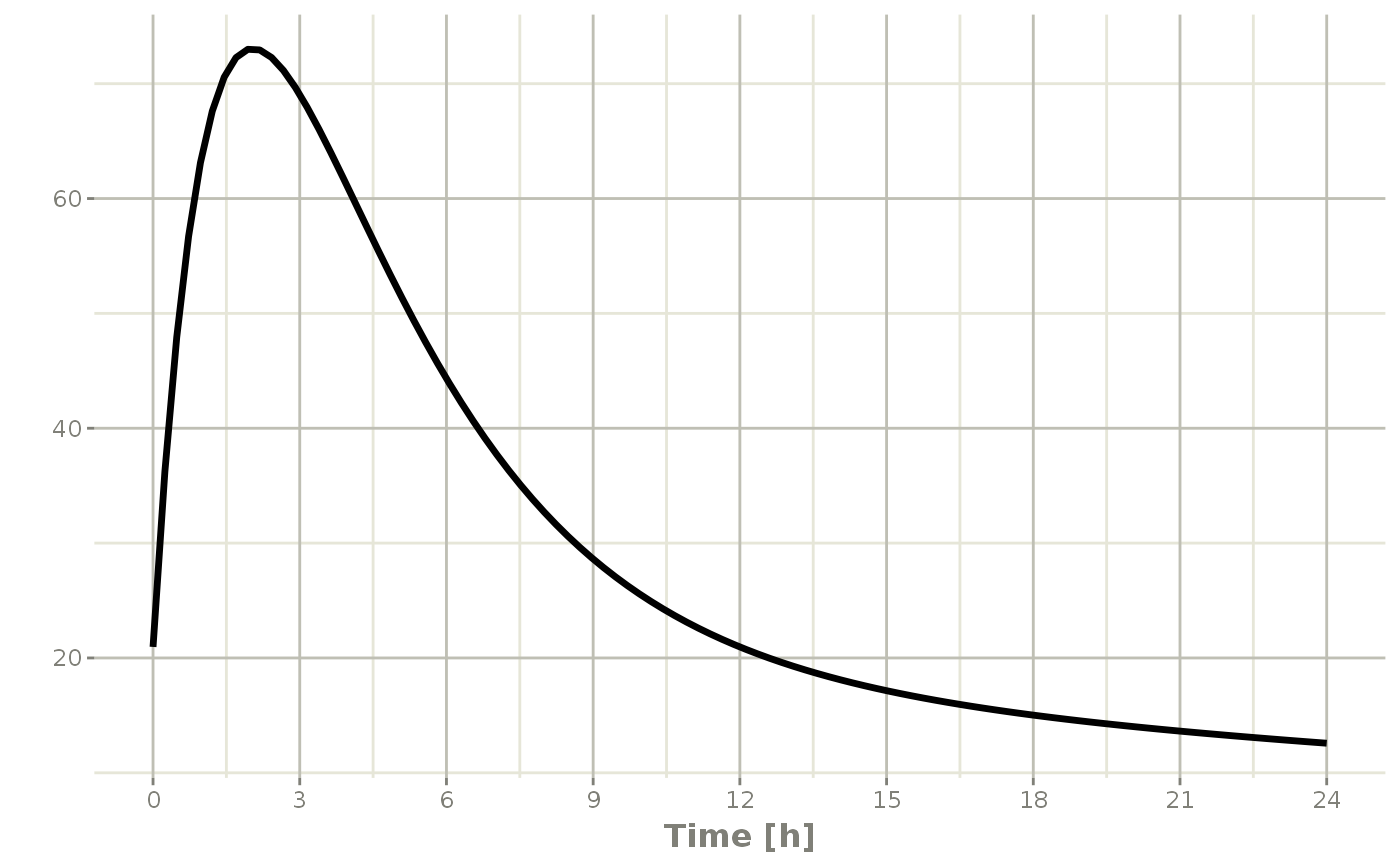Steady state for complex dosing By using the ss=2 flag, you can use the super-positioning principle in linear kinetics to get steady state nonstandard dosing (i.e. morning 100 mg vs evening 150 mg). This is done by: • Saving all the state values • Resetting all the states and solving the system to steady state • Adding back all the prior state values ev <- et(timeUnits="hr") %>% et(amt=10000, ii=24, ss=1) %>% et(time=12, amt=15000, ii=24, ss=2) %>% et(time=24, amt=10000, ii=24, addl=3) %>% et(time=36, amt=15000, ii=24, addl=3) %>% et(seq(0, 64, length.out=500)) library(ggplot2) rxSolve(m1, ev,maxsteps=10000) %>% plot(C2) + annotate("rect", xmin=0, xmax=24, ymin=-Inf, ymax=Inf, alpha=0.2) + annotate("text", x=12.5, y=7, label="Initial Steady State Period") + annotate("text", x=44, y=7, label="Steady State AM/PM dosing")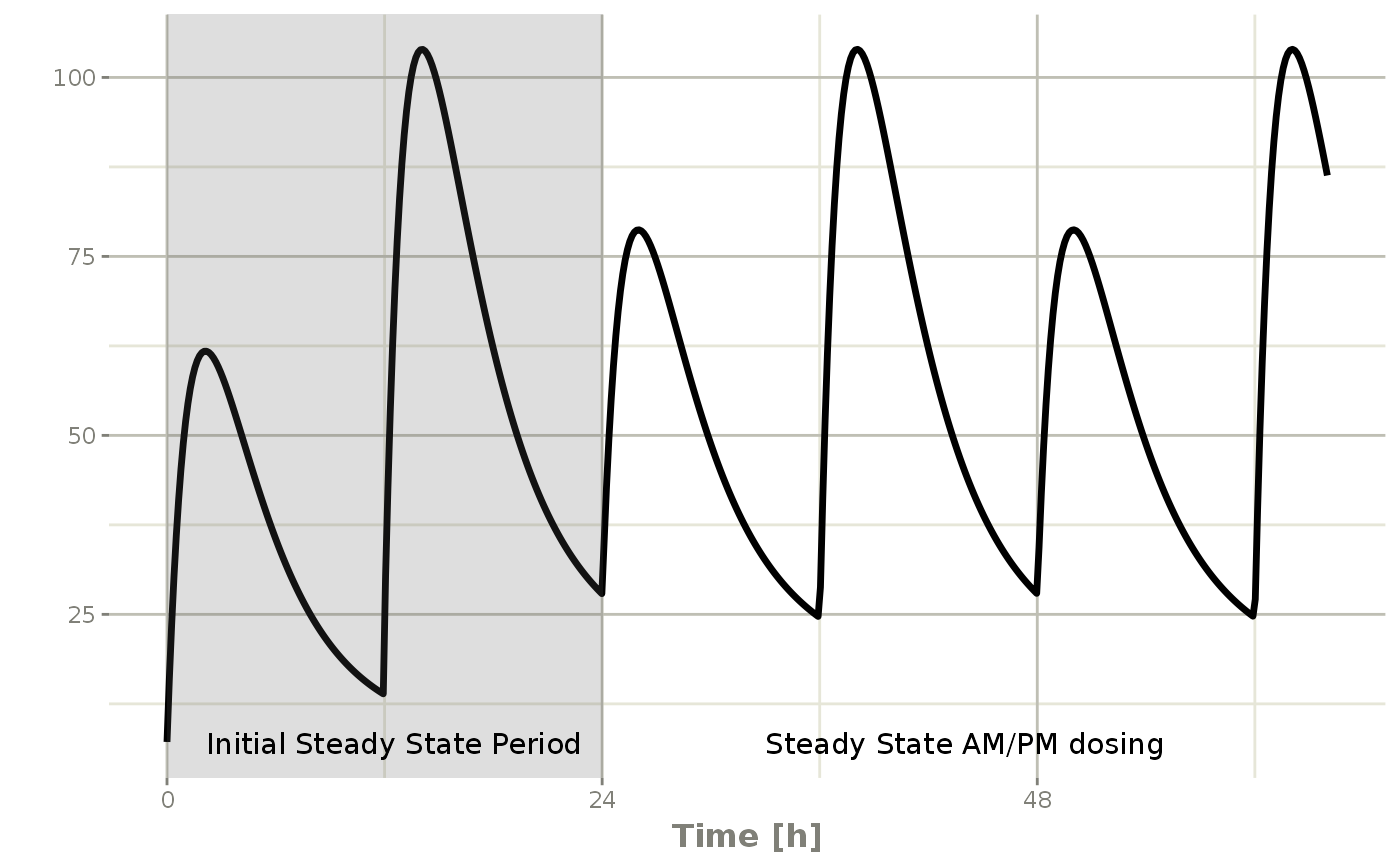You can see that it takes a full dose cycle to reach the true complex steady state dosing. Steady state for constant infusion or zero order processes The last type of steady state that RxODE supports is steady-state constant infusion rate. This can be specified the same way as NONMEM, that is: • No inter-dose interval ii=0 • A steady state dose, ie ss=1 • Either a positive rate (rate>0) or a estimated rate rate=-1. • A zero dose, ie amt=0 • Once the steady-state constant infusion is achieved, the infusion is turned off when using this record, just like NONMEM. Note that rate=-2 where we model the duration of infusion doesn’t make much sense since we are solving the infusion until steady state. The duration is specified by the steady state solution. Also note that bioavailability changes on this steady state infusion also do not make sense because they neither change the rate or the duration of the steady state infusion. Hence modeled bioavailability on this type of dosing event is ignored. Here is an example: ev <- et(timeUnits="hr") %>% et(amt=0, ss=1,rate=10000/8) p1 <- rxSolve(m1, ev) %>% plot(C2, eff) ev <- et(timeUnits="hr") %>% et(amt=200000, rate=10000/8) %>% et(0, 250, length.out=1000) p2 <- rxSolve(m1, ev) %>% plot(C2, eff) library(patchwork) p1 / p2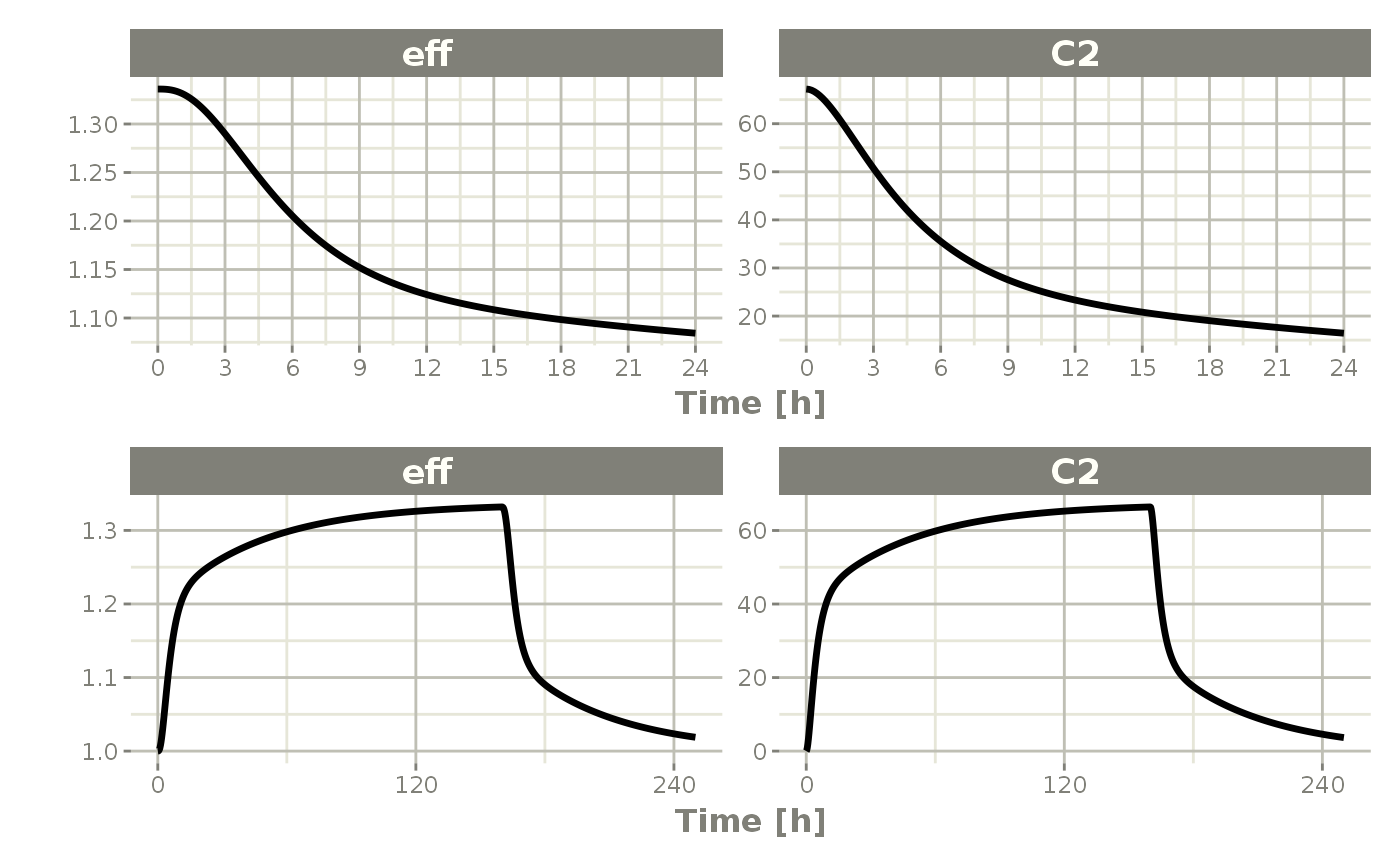Not only can this be used for PK, it can be used for steady-state disease processes. Reset Events Reset events are implemented by evid=3 or evid=reset, for reset and evid=4 for reset and dose. ev <- et(timeUnits="hr") %>% et(amt=10000, ii=12, addl=3) %>% et(time=6, evid=reset) %>% et(seq(0, 24, length.out=100)) ev #> -------------------------- EventTable with 102 records ------------------------- #> #> 2 dosing records (see x$get.dosing(); add with add.dosing or et)
#>    100 observation times (see x$get.sampling(); add with add.sampling or et) #> multiple doses in addl columns, expand with x$expand(); or etExpand(x)
#> -- First part of x: ------------------------------------------------------------
#> # A tibble: 102 x 5
#>     time   amt    ii  addl evid
#>      [h] <dbl>   [h] <int> <evid>
#>  1 0        NA    NA    NA 0:Observation
#>  2 0     10000    12     3 1:Dose (Add)
#>  3 0.242    NA    NA    NA 0:Observation
#>  4 0.485    NA    NA    NA 0:Observation
#>  5 0.727    NA    NA    NA 0:Observation
#>  6 0.970    NA    NA    NA 0:Observation
#>  7 1.21     NA    NA    NA 0:Observation
#>  8 1.45     NA    NA    NA 0:Observation
#>  9 1.70     NA    NA    NA 0:Observation
#> 10 1.94     NA    NA    NA 0:Observation
#> # ... with 92 more rows

The solving show what happens in this system when the system is reset at 6 hours post-dose.

rxSolve(m1, ev) %>% plot(depot,C2, eff)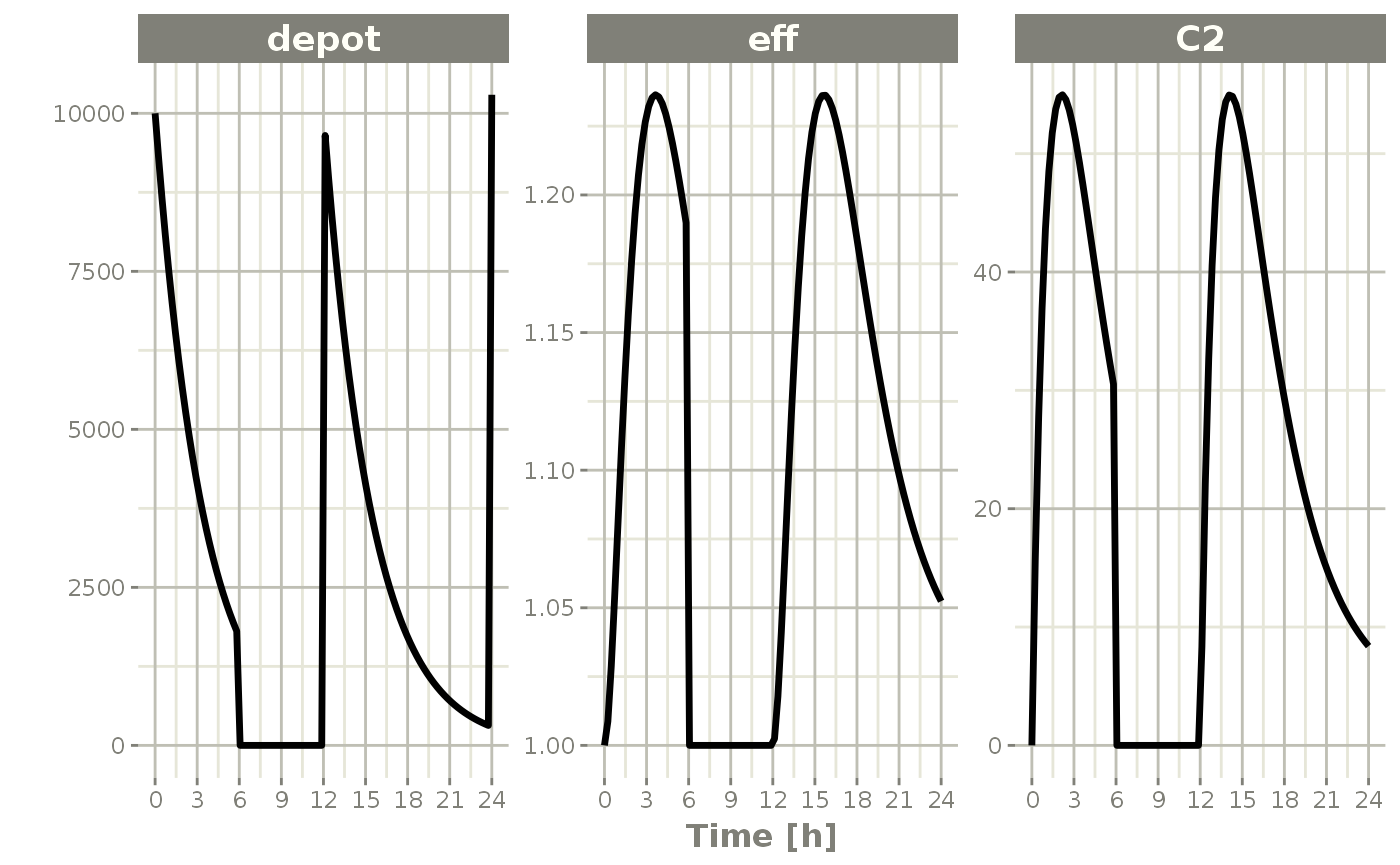You can see all the compartments are reset to their initial values. The next dose start the dosing cycle over.

ev <- et(timeUnits="hr") %>%
et(time=6, amt=10000, evid=4) %>%
et(seq(0, 24, length.out=100))

ev
#> -------------------------- EventTable with 102 records -------------------------
#>
#>    2 dosing records (see x$get.dosing(); add with add.dosing or et) #> 100 observation times (see x$get.sampling(); add with add.sampling or et)
#>    multiple doses in addl columns, expand with x$expand(); or etExpand(x) #> -- First part of x: ------------------------------------------------------------ #> # A tibble: 102 x 5 #> time amt ii addl evid #> [h] <dbl> [h] <int> <evid> #> 1 0 NA NA NA 0:Observation #> 2 0 10000 12 3 1:Dose (Add) #> 3 0.242 NA NA NA 0:Observation #> 4 0.485 NA NA NA 0:Observation #> 5 0.727 NA NA NA 0:Observation #> 6 0.970 NA NA NA 0:Observation #> 7 1.21 NA NA NA 0:Observation #> 8 1.45 NA NA NA 0:Observation #> 9 1.70 NA NA NA 0:Observation #> 10 1.94 NA NA NA 0:Observation #> # ... with 92 more rows In this case, the whole system is reset and the dose is given rxSolve(m1, ev) %>% plot(depot,C2, eff)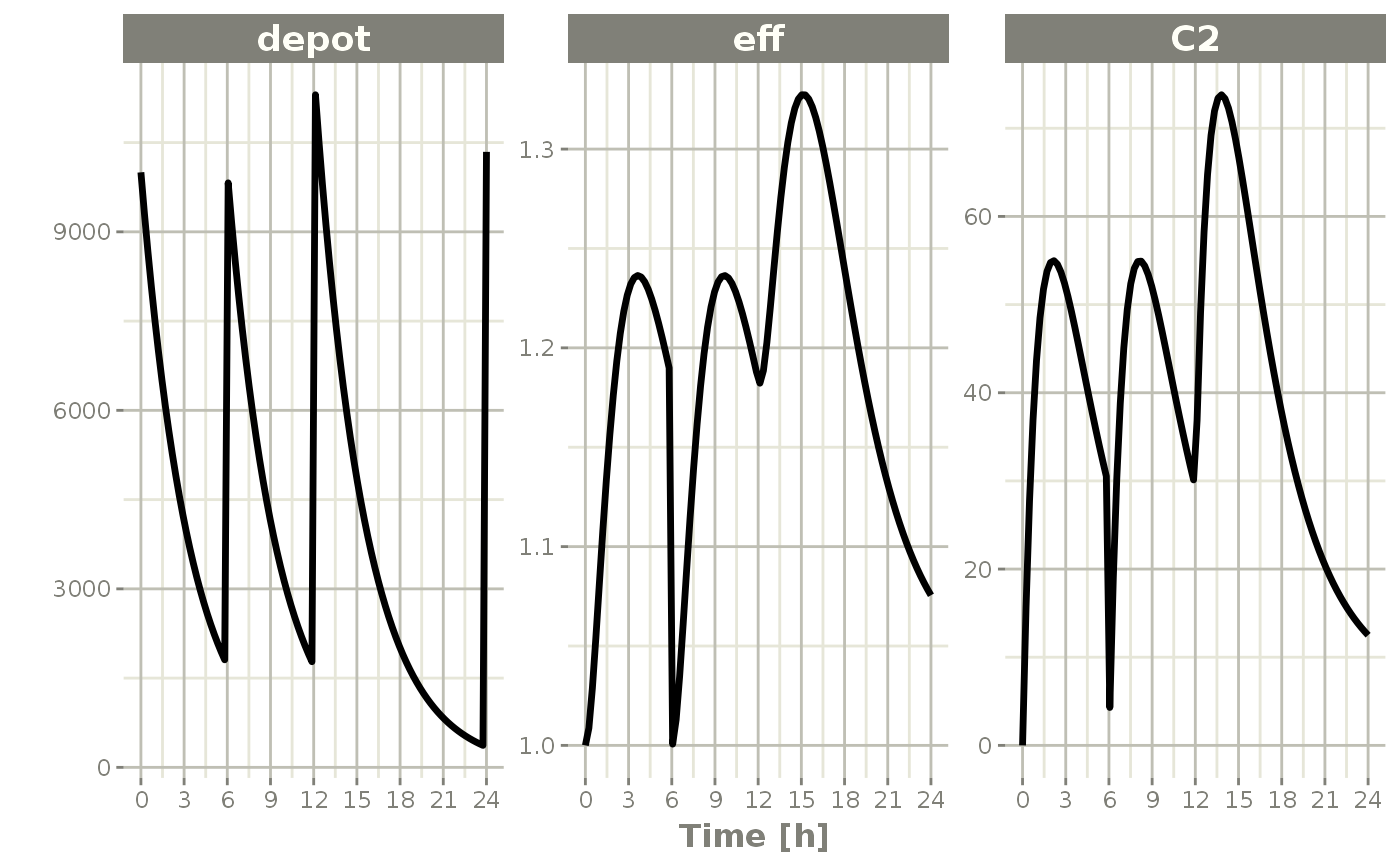Turning off compartments You may also turn off a compartment, which is similar to a reset event. ev <- et(timeUnits="hr") %>% et(amt=10000, ii=12, addl=3) %>% et(time=6, cmt="-depot", evid=2) %>% et(seq(0, 24, length.out=100)) ev #> -------------------------- EventTable with 102 records ------------------------- #> #> 2 dosing records (see x$get.dosing(); add with add.dosing or et)
#>    100 observation times (see x$get.sampling(); add with add.sampling or et) #> multiple doses in addl columns, expand with x$expand(); or etExpand(x)
#> -- First part of x: ------------------------------------------------------------
#> # A tibble: 102 x 6
#>     time cmt         amt    ii  addl evid
#>      [h] <chr>     <dbl>   [h] <int> <evid>
#>  1 0     (obs)        NA    NA    NA 0:Observation
#>  2 0     (default) 10000    12     3 1:Dose (Add)
#>  3 0.242 (obs)        NA    NA    NA 0:Observation
#>  4 0.485 (obs)        NA    NA    NA 0:Observation
#>  5 0.727 (obs)        NA    NA    NA 0:Observation
#>  6 0.970 (obs)        NA    NA    NA 0:Observation
#>  7 1.21  (obs)        NA    NA    NA 0:Observation
#>  8 1.45  (obs)        NA    NA    NA 0:Observation
#>  9 1.70  (obs)        NA    NA    NA 0:Observation
#> 10 1.94  (obs)        NA    NA    NA 0:Observation
#> # ... with 92 more rows

Solving shows what this does in the system:

rxSolve(m1, ev) %>% plot(depot,C2, eff)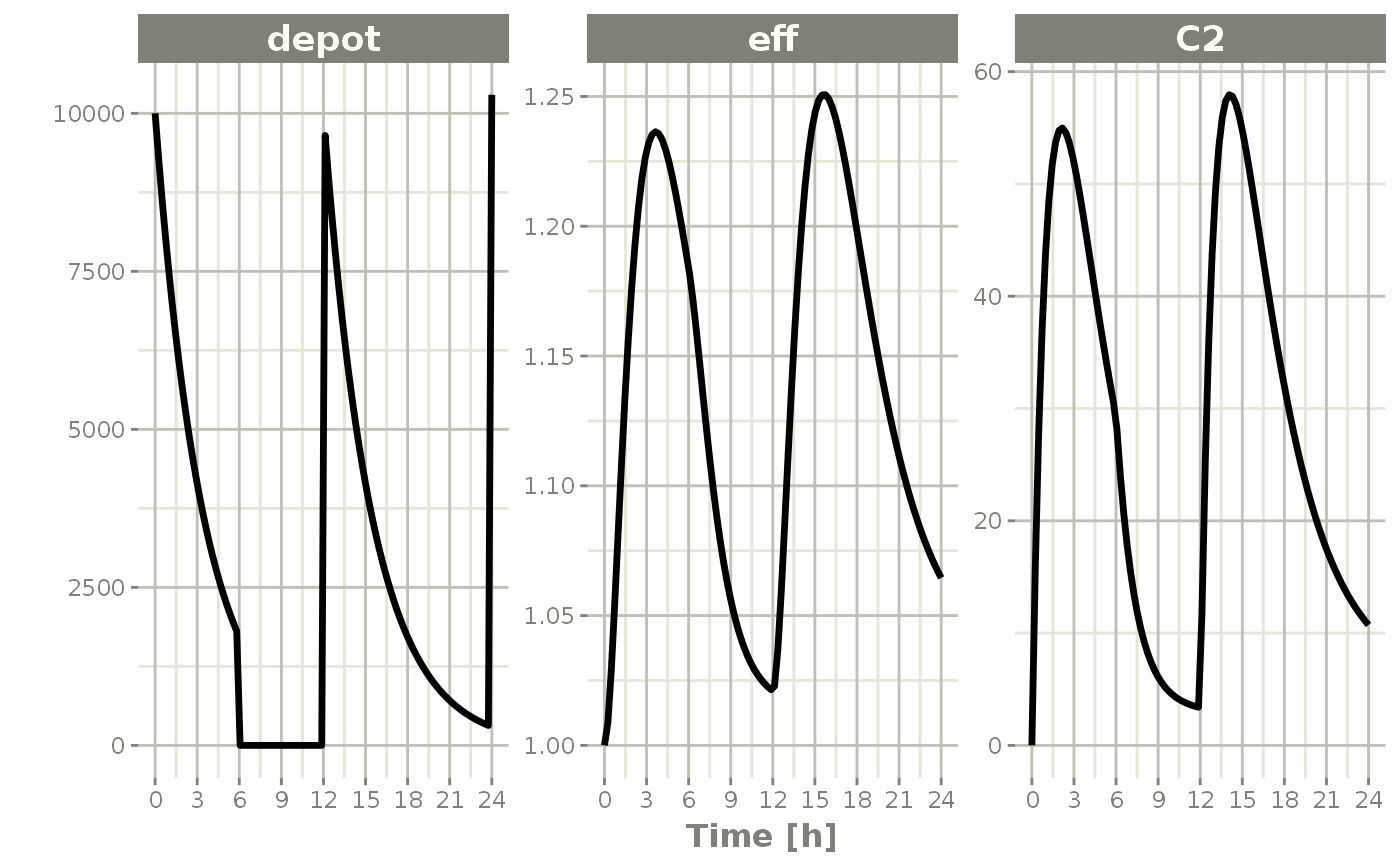In this case, the depot is turned off, and the depot compartment concentrations are set to the initial values but the other compartment concentrations/levels are not reset. When another dose to the depot is administered the depot compartment is turned back on.

Note that a dose to a compartment only turns back on the compartment that was dosed. Hence if you turn off the effect compartment, it continues to be off after another dose to the depot.

ev <- et(timeUnits="hr") %>%
et(time=6, cmt="-eff", evid=2) %>%
et(seq(0, 24, length.out=100))

rxSolve(m1, ev) %>% plot(depot,C2, eff)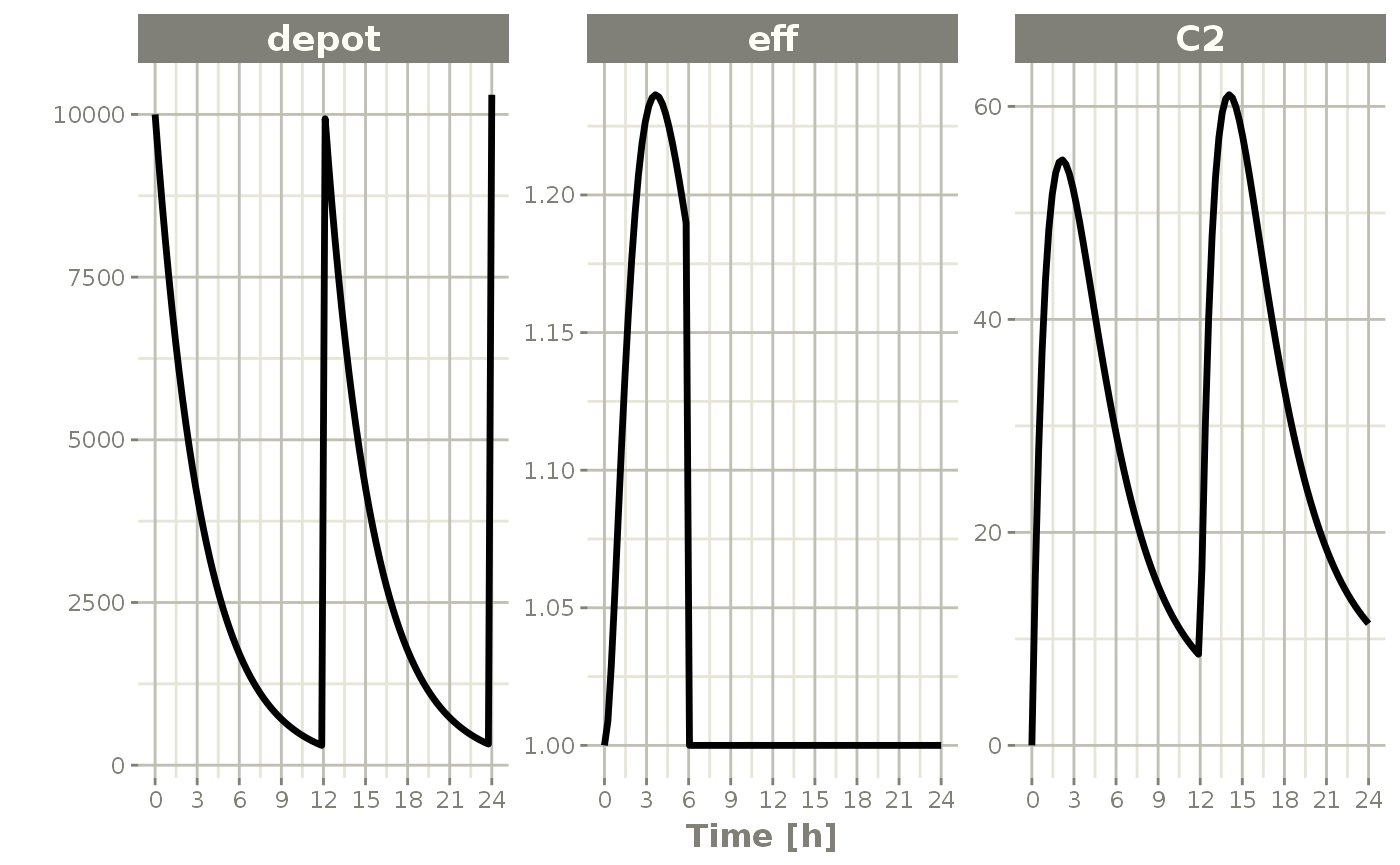To turn back on the compartment, a zero-dose to the compartment or a evid=2 with the compartment would be needed.

ev <- et(timeUnits="hr") %>%